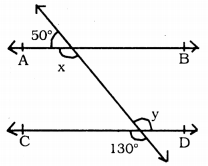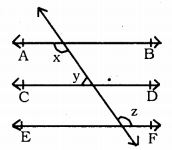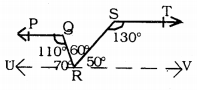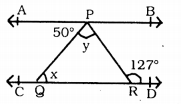# KSEEB Solutions for Class 9 Maths Chapter 3 Lines and Angles Ex 3.2

KSEEB Solutions for Class 9 Maths Chapter 3 Lines and Angles Ex 3.2 are part of KSEEB Solutions for Class 9 Maths. Here we have given Karnataka Board Class 9 Maths Chapter 3 Lines and Angles Exercise 3.2.

## Karnataka Board Class 9 Maths Chapter 3 Lines and Angles Ex 3.2

Question 1.
In Fig. 3.28. find the values of x and y and then shows that AB||CD.i) ∠x = ?
ii) ∠y = ?
iii) Show tht AB||CD.(i) PQ Bisects AB at R.
∠PRA and ∠ARS are on straight line PQ.
∴ ∠PRA + ∠ARS = 180° Adjacent angles
50 + ∠ARS = 180°
∠ARS = 180 – 50
∴ x = ∠ARS = 130° (i)

(ii) Similarly, PQ straight line intersects straight line CD at S.
∴ ∠CSQ = ∠RSD . Vertically opposite angles
∴ y = ∠RSD = 130° (ii)
From (i) and (ii),
∠ARS = ∠RYD
x = y = 130°

(iii) Therse are mutual alternate angles
∴ x = y = 130°
∴ AB || CD.

Question 2.
In Fig. 3.29, if AB || CD, CD || EF and y : z = 3 : 7, find x.PQ straight line intersects AB||CD]|EF at points R, S, T.
If y : z = 3 : 7, then value of ‘x’ = ?
PQ straight line intersects CD||EF at S and T.
∴ ∠CSR = ∠DST = y (∵ vertically opposite angle)
But, ∠DST + ∠STF = 180°
(∵ Sum of interior angles in the same side)
∴ y + z = 180°
But, y : z = 3 : 7.
Sum of ratio 3 + 7 = 10
If ratio 10 means 180
If ratio 9 means ?
$$\frac{180 \times 3}{10}=54^{\circ}$$
∴ y = 54°
y + z = 180
54 + z = 180
∴ z = 180 – 54
∴ z = 126°
But, x + y = 180
(∵ Sum of interior angles on one side)
x + 54 = 180
∵ x = 180 – 54
∵ x = 126°.

Question 3.
In Fig. 3.30, If AB||CD, EF ⊥ CD and ∠GED = 126°, find ∠AGE, ∠GEF and ∠FGE.AB||CD and EF⊥CD and ∠GED = 126°∠AGE =?
∠GEF =?
∠FGE =?
∠GED = 126°.
∴ ∠GEF + ∠ZFED = 126°
∠GEF + 90° = 126° ∵ EF ⊥ CD.
∴ GEF = 126-90
∴  GEF = 36°.
∴ ∠GFE = 90° . EF ⊥ AB
∴ In ∆GEF
∠FGE + ∠GFE + ∠GEF = 180°
∠FGE + 90° + 36° = 180°
∠FGE + 126° = 180°
∴ ∠FGE = 180-126
∴ ∠FGE = 54°.
∠AGE + ∠FGE = 180° (Adjacent angles)
∠AGE + 54° = 180°
∴ ∠AGE =180-54
∠AGE = 126°
∴ ∠AGE = 126°
∠GEF = 36°
∠FGE = 54°.

Question 4.
In Fig. 3.31, If PQ||ST, ∠PQR =110°, and ∠RST = 130°, find ∠QRS.
(Hint: Draw a line parallel to ST through point R).Data: PQ||ST and ∠PQR= 110° and ∠RST= 130°.
To Prove: ∠QRS =?
Construction: Draw ST||UV through ‘R’
Proof: PQ||ST (Data)
ST || UV (Construction)
∴ PQ||ST||UV
PQ||UV.
∴ ∠PQR + ∠URQ = 180° (Sum of interior angles)
110 + ∠URQ = 180°
∠URQ = 180- 110
∴ ∠URQ = 70° (i)
Similarly, ST||UV.
∴ ∠RST + ∠SRV = 180°
130 + ∠SRV = 180
∴ ∠SRV = 180 – 130
∴ ∠SRV = 50° (ii)
But, URV is a straight line.
∴ ∠URV = 180°.
∠URQ + ∠QRS + ∠RSV = 180°
70 + ∠QRS + 50 = 180
∠QRS + 120 = 180°
∴ ∠QRS = 180 – 120
∴ ∠QRS = 60°.

Question 5.
In Fig. 3.32. if AB||CD, ∠APQ = 50° and ∠PRD = 127°, find x and y.If AB || CD and ∠APQ= 50° and ∠PRD = 127°, then ∠x = ? ∠y = ?
AB || CD PQ straight line intersects these at P and Q.
∴ ∠APQ = ∠PQR . alternate angles.
50 = ∠PQR = x
∴ x = 50°
Similarly, AB || CD PR straight line intersects these at P and R.
∴ ∠APR = ∠PRD
∠APQ + ∠QPR = ∠PRD
50 + ∠QPR = 127
∠QPR = 127 – 50
∴∠QPR = 77 °
∠QPR = y = 77 °
∴ x = 50 °,
y = 77 °.

Question 6.
In Fig. 3.33, PQ and RS are two mirrors placed parallel to each other. An incident ray AB strikes the mirror PQ at B, the reflected ray moves along the path BC and strikes the mirror RS at C and again reflects back along CD.
Prove that AB||CD.